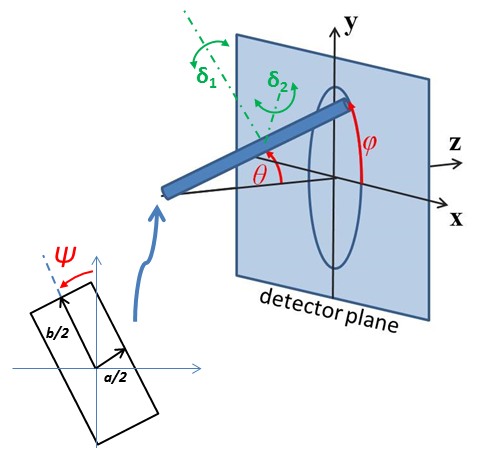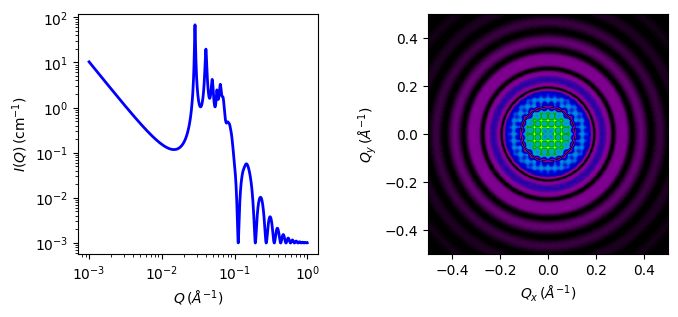# sc_paracrystal

Simple cubic lattice with paracrystalline distortion

Parameter

Description

Units

Default value

scale

Scale factor or Volume fraction

None

1

background

Source background

cm-1

0.001

dnn

Nearest neighbor distance

220

d_factor

Paracrystal distortion factor

None

0.06

40

sld

Sphere scattering length density

10-6-2

3

sld_solvent

Solvent scattering length density

10-6-2

6.3

theta

c axis to beam angle

degree

0

phi

degree

0

psi

degree

0

The returned value is scaled to units of cm-1 sr-1, absolute scale.

Warning

This model and this model description are under review following concerns raised by SasView users. If you need to use this model, please email help@sasview.org for the latest situation. The SasView Developers. September 2018.

Definition

Calculates the scattering from a simple cubic lattice with paracrystalline distortion. Thermal vibrations are considered to be negligible, and the size of the paracrystal is infinitely large. Paracrystalline distortion is assumed to be isotropic and characterized by a Gaussian distribution.

The scattering intensity $$I(q)$$ is calculated as

$I(q) = \text{scale}\frac{V_\text{lattice}P(q)Z(q)}{V_p} + \text{background}$

where scale is the volume fraction of spheres, $$V_p$$ is the volume of the primary particle, $$V_\text{lattice}$$ is a volume correction for the crystal structure, $$P(q)$$ is the form factor of the sphere (normalized), and $$Z(q)$$ is the paracrystalline structure factor for a simple cubic structure.

Equation (16) of the 1987 reference is used to calculate $$Z(q)$$, using equations (13)-(15) from the 1987 paper for $$Z1$$, $$Z2$$, and $$Z3$$.

The lattice correction (the occupied volume of the lattice) for a simple cubic structure of particles of radius R and nearest neighbor separation D is

$V_\text{lattice}=\frac{4\pi}{3}\frac{R^3}{D^3}$

The distortion factor (one standard deviation) of the paracrystal is included in the calculation of $$Z(q)$$

$\Delta a = gD$

where g is a fractional distortion based on the nearest neighbor distance.

The simple cubic lattice is

For a crystal, diffraction peaks appear at reduced q-values given by

$\frac{qD}{2\pi} = \sqrt{h^2+k^2+l^2}$

where for a simple cubic lattice any h, k, l are allowed and none are forbidden. Thus the peak positions correspond to (just the first 5)

Note

The calculation of Z(q) is a double numerical integral that must be carried out with a high density of points to properly capture the sharp peaks of the paracrystalline scattering. So be warned that the calculation is slow. Fitting of any experimental data must be resolution smeared for any meaningful fit. This makes a triple integral which may be very slow.

The 2D (Anisotropic model) is based on the reference below where I(q) is approximated for 1d scattering. Thus the scattering pattern for 2D may not be accurate particularly at low $$q$$. For general details of the calculation and angular dispersions for oriented particles see Oriented particles. Note that we are not responsible for any incorrectness of the 2D model computation.Fig. 57 Orientation of the crystal with respect to the scattering plane, when $$\theta = \phi = 0$$ the $$c$$ axis is along the beam direction (the $$z$$ axis).Fig. 58 1D and 2D plots corresponding to the default parameters of the model.

Source

sc_paracrystal.py $$\ \star\$$ sc_paracrystal.c $$\ \star\$$ gauss150.c $$\ \star\$$ sphere_form.c $$\ \star\$$ sas_3j1x_x.c

Reference

Authorship and Verification

• Author: NIST IGOR/DANSE Date: pre 2010